# AP Board 9th Class Physical Science Solutions Chapter 9 Floating Bodies

AP State Syllabus AP Board 9th Class Physical Science Solutions Chapter 9 Floating Bodies Textbook Questions and Answers.

## AP State Syllabus 9th Class Physical Science Solutions 9th Lesson Floating Bodies

### 9th Class Physical Science 9th Lesson Floating Bodies Textbook Questions and Answers

Question 1.
A solid sphere has a radius of 2 cm and a mass of 0.05 kg. What is the relative density of the sphere? (AS 1)
Radius of the sphere = 2 cm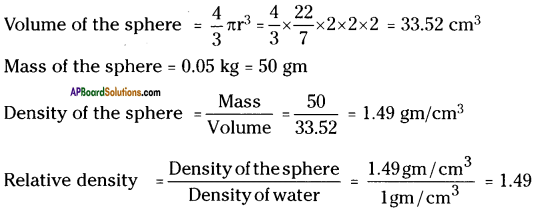Question 2.
A small bottle weighs 20 g when empty and 22 g when filled with water. When it is filled with oil it weighs 21.76 g. What is the density of oil? (AS 1)
Weight of water = 22 – 20 = 2 gm
Weight of oil = 21.76 – 20 = 1.76 gm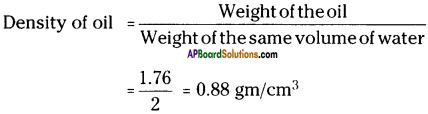Question 3.
An ice cube floats on the surface of a glass of water (density of ice = 0.9 g/cm3). When the ice melts will the water level in the glass rise? (AS 1)
Yes, the water level rises.

Reason :
The ice cube floats on water, because its density is less than the density of water. When ice cube melts, it becomes water, so that the water level rises.Question 4.
The volume of 50 g of a substance is 20 cm³. If the density of water is 1 g/cm³, will the substance sink or float when placed on the surface of water? What will be the mass of water displaced by the substance? (AS 1)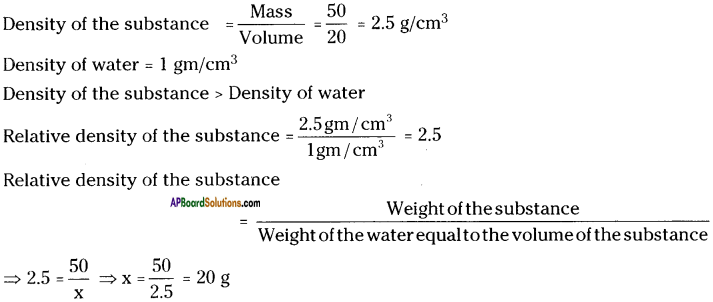∴ Weight of the water displaced by the substance = 20 g

Question 5.
Find the pressure at a depth of 10 m in water if the atmospheric pressure is 100 kPa. [1Pa = 1 N/m²] [100kPa = 105 Pa = 105 N/m² = 1 atm.] (AS 1)
Depth ‘h’ = 10 m ; Atmospheric pressure P0 = 100 kPa
Density of water p = 1 gm/cm³ = 1 kg/m³
Pressure at a depth ‘h’ is P = P0 + hρg
= 100 + 10 x 1 x 9.8
= 100 + 98
= 198 kPa

Question 6.
Why do some objects float on the water? And some sink? (AS 1)

• Floating or sinking of objects on water depends on two factors.
a) Relative density
b) Weight of the water displace by the object
• If the relative density of an object is greater than 1, the object sinks otherwise it floats.
• Eventhough the relative density is greater than 1, if the weight of the water displaced by the object equal to the weight of the object itself, the object floats on the water.Question 7.
Explain density and relative density and write their formulae. (AS 1)
Density :
Density is defined as mass per unit volume.
Density = $$\frac{\text { Mass }}{\text { Volume }}$$

Unit of density is gm/cm³ or kg/m³.

Relative density :
Relative density of an object is the ratio of density of the object to the density of water.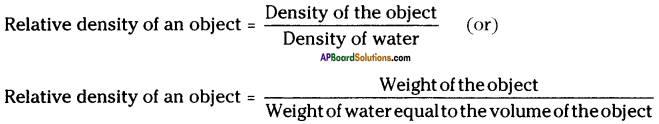Question 8.
What is the value of density of water? (AS 1)
Value of density of water = 1 gm/cm³ (or) 1 kg/cm³.

Question 9.
Find the relative density of wood. Explain the process. (Lab Activity 1) (AS 3)
Aim :
To find the relative density of wood.

Materials required :
Overflow vessel, 50 ml measuring cylinder, spring balance, wooden block, water.Procedure:

1. Weigh the 50 ml measuring cylinder and note its weight.
2. Weigh the wooden block and note its weight.
3. Pour water in the overflow vessel until it starts dripping from its beak.
4. When water stops dripping from the beak, place the 50 ml measuring cylinder under it.
5. Slip the wooden block gently into the overflow vessel, ensuring that the water does not splash out.
6. Once the wooden block is in the overflow vessel, water flows out of the beak and collects in the 50 ml cylinder.
7. Wait till the flow of water from beak, stops.
8. Weigh the cylinder with the water that overflowed and record the weight.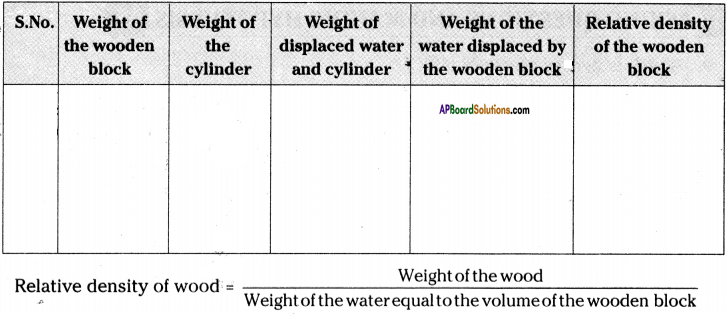Question 10.
Which is denser, water or milk? (AS 2)
The density of water is 1 gm/cc and that of milk is 1.02 gm/cc. Hence milk is slightly denser than water.Question 11.
What is buoyancy? (AS 1)
Buoyancy is the upward force that a fluid exerts on an object less denser than itself.
(or)
Buoyancy is the ability of an object to float in a liquid.

Question 12.
Classify the following things into substances having relative density > 1 and relative density < 1. Wood, iron, rubber, plastic, glass, stone, cork, air, coal, ice, wax, paper, milk, kerosene, groundnut oil, soap. (AS 1)

 Relative density > 1 Relative density < 1 Iron Wood Glass Rubber Stone Plastic Milk Cork Soap Air Coal Ice Wax Paper Kerosene Groundnut oil

Question 13.
How can you appreciate the technology of making ships float, using the material which sink in water? (AS 6)

• We know that a piece of iron sinks in water.
• The relative density of iron is 8.5 which is many times more than water.
• A ship that floats on water is made up of tonnes of iron. It is really wonderful.
• According to the Archimedes principle of buoyancy, any object can float when its weight is equal to the weight of water displaced by it.
• Hence ships are made with a larger surface area so that it displaces the water, whose weight is equal to the weight of loaded ship.
• It involves very sharp scientific calculations and a large engineering technology.
• Really such type of technologies are highly appreciable and the scientists who formulates all these are also really great.

Question 14.
Can you make iron float? How? (AS 3)
Yes, we can make iron to float on water.
Procedure:

1. Take a piece of iron and drop it in a vessel of water.
2. We observe that the iron piece sinks in water.
3. Take a thin foil of iron and fold it into four folds.
4. Drop it in water. It sinks.
5. Now unfold the foil and bend it in the form of a bowl. [You can use an iron tin also]
6. Now drop the bowl in water.
7. If floats on water.

Reason:
The weight of water displaced by iron bowl (iron tin) is less than the weight of the iron bowl (iron tin).Question 15.
How can you find the relative density of a liquid? (Lab Activity – 2) (AS 3)
Aim :
To find the relative density of a liquid.

Materials required :
Small bottle of 50 ml capacity (the bottle should weigh not less than 10 gm), spring balance, any liquid (milk or oil or kerosene) about 50 ml.

Procedure:

1. Find the weight of empty 50 ml bottle.
2. Fill the bottle with water and weigh it.
3. Find the weight of 50 ml water.
4. Remove water from the bottle and fill it with any liquid (say milk).
5. Weigh the bottle with liquid.
6. Weight of 50 ml liquid = Weight of bottle with liquid – Weight of empty bottle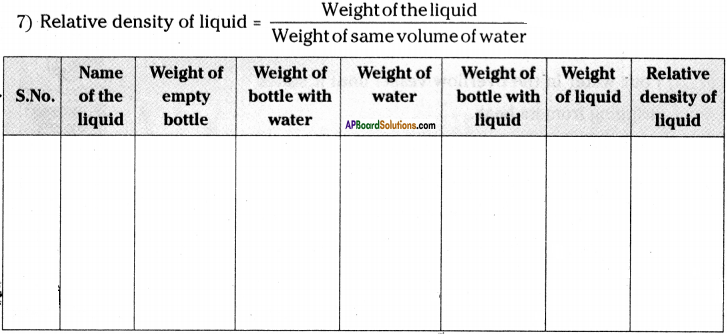Question 16.
Find the relative density of different fruits and vegetables and make a list. (AS 3)

• Follow the procedure in Q.No (11) of A.S – (3).
• Use different fruits and vegetables in the place of wooden block.
• Write the observed values in the following table.
 Name of the fruit or vegetable Relative density Cabbage 0.36 Cauliflower 0.26 Bottleguard 0.56 Potato 0.67 Onion 0.59 Chilli 0.29 Bitterguard 0.4 Apple 1.22 Grape 1.04 Orange 0.34

Question 17.
Make a lactometer with ball point refill. What would you do to make the refill stand vertically straight? (Activity – 2) (AS 5)• Take an empty ball pen refill. It should have a metal point.
• Take a boiling tube and fill it with water.
• Put the refill in with metallic point inside the water.
• Use a pen to mark the point on the refill to show the part which is above the water surface.
• Pour out the water from the boiling tube and fill it with milk.
• Float the refill in the milk.
• Puf the record mark on the refill, at the point showing the part which is above the surface of the milk.
• These two marks are not at the same place. This is the improvised lactometer.
• We have to attach a small weight at the bottom of the refill to make it stand vertically straight.

Question 18.
Draw the diagram of a mercury barometer. (AS 5)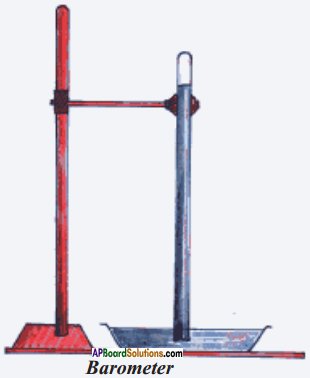Question 19.
Write a note on Pascal’s discovery in helping to make hydraulic jacks. (AS 6)
Pascal’s principle:
External pressure applied to an enclosed body of fluid is transmitted equally in all directions throughout the fluid volume and the walls of the containing vessel.

Use :

1. This principle is used in designing and working of hydraulic jacks.
2. Hydraulic jacks are useful to lift heavy objects like cars and other vehicles in automobile work shops when the vehicles are to be required or repaired.
3. Here very less force is used to lift such heavy vehicles.

Appreciation :

1. Hydraulic jacks are used not only in automobile work shops, but also in so many industries where heavy weights are to be lifted with a little force.
2. All the comforts which we are enjoying now are the efforts of scientists who discovered the laws and principles.
3. Hence we have to appreciate the efforts of Pascal for his contribution in designing these principles.Question 20.
Write a note on Archimedes discovery of force of buoyancy. (AS 6)
Archimedes principle:
When a body is immersed in a fluid, it experiences an upward force of buoyancy equal to the weight of fluid displaced by the immersed portion of the body.

Use :
The principle is used to determine the purity of metals.

Appreciation :

1. This principle was discovered by Archimedes eventually when he was taking bath.
2. With this principle he could solve the problem assigned by the king to him.
3. So many problems in our life may be solved by so many scientific principles discovered by various scientists.
4. You might have heard about falling of the statue of Budha in Hussain Sagar.
5. That statue was lifted by using the principle of buoyancy.
6. Archimedes is thought to be so important as a mathematician that scientists honoured him.
a) A large hole or crator on the moon is named after Archimedes.
b) Some mountains on the moon are called the monte – Archimedes.
7. Hence the efforts of Archimedes in discovering such type of principle may be highly appreciated.

Question 21.
You found the relative densities of some solids and some liquids by doing some activities. List the solids and liquids in increasing order of relative density. (AS 4)

 Substance Relative density Kerosene 0.81 Rubber 0.94 Milk 1.02 Glass 1.29 Iron 8.5

Question 22.
Iron sinks in water, wood floats in water. If we tie an iron piece to wood piece of the same volume, buritlle and drop it in water, would bundle sink or float? Make a guess and find out whether your guess is correct or wrong with an experiment. Give reasons. (AS 2, AS 3)
The body sinks in water.

Reason :
The combined mass of the system increases, so the combined density also increases. Hence the body sinks in water.

Question 23.
Air brakes in automobiles work on Pascal’s principle. What about air brakes? Collect the information about the working process of air brakes. (AS 4)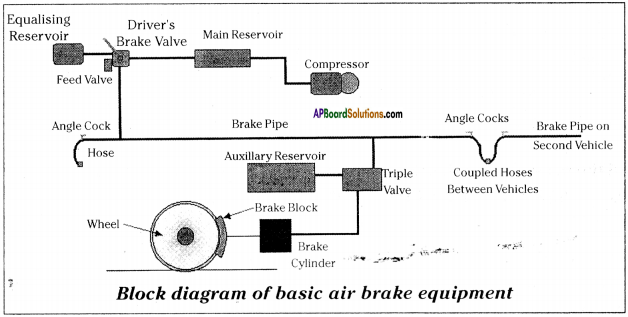• Air brakes works on the principle of conversion of energy. Generally in trains, while it is moving -fit .produces kinetic energy. This kinetic energy has to be reduced to make the train stop. Here air is used to reduce kinetic energy by converting it into heat energy.
• The system of air brakes in trains has been shown in the figure.
• The important parts are compressor, main reservoir, driver’s brake value, brake pipe, triple value, auxiliary reservoir, brake cylinders, and brake block.

Working:

1. When the driver placed the value in application position, the air pressure in the brake pipe escapes.
2. The loss of pressure is detected by the slide value in the triple value.
3. Now a connection between the auxiliary reservoir and the brake cylinder has been opened and the air in the auxiliary reservoir feeds through into the brake cylinder.
4. The air pressure forces the piston to move against the spring pressure and causes the brakes to be applied to the wheels.Question 24.
Where do you observe Archimedes principle in daily life? Give two examples.
Daily life application of Archimedes principle :

1. Archimedes principle of buoyancy is applied in our daily life in many ways.
2. Fish, human swimmers, ice bergs and ships float follow Archimedes principle of buoyancy.
3. Rise of balloon in air also follows Archimedes principle.
4. While dragging water from a well, the bucket filled with water seems to be weightless till it reaches the surface of the water in the well. This is also due to buoyancy.
5. Swimming of duck in water is also an example of Archimedes principle.

Question 25.
Where do you observe Pascal’s principle in daily life? Give a few examples.
(OR)
Write any one application of Pascal’s principle in daily life.
Daily life application of Pascal’s principle :
Pascal’s principle is applied in the working of

1. Hydraulic jacks
2. Hydraulic lifts
3. Hydraulic pumps
4. Hydraulic cranes
5. Siphons
6. Artesian wells
7. Water towers and dams

### 9th Class Physical Science 9th Lesson Floating Bodies InText Questions and Answers

9th Class Physical Science Textbook Page No. 141

Question 1.
a) Did kerosene float above the water or did water float above the kerosene?
Kerosene floats above the water.

b) Which objects float in kerosene?
Plastic buttons, match stick, tiny paper balls, wax, etc. floats in kerosene.

c) Which objects sink in kerosene but float on water?
Wax sink in kerosene but float on water.

d) Which objects sink in water?
Pins, small pebbles, sand, etc. sink in water.

e) Draw a diagram of the tube, showing the results of your activity.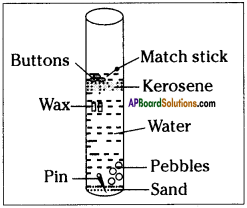f) Why did different objects behave differently?
The density of objects is the main reason to behave differently.

9th Class Physical Science Textbook Page No. 144

Question 2.
1) What is the relative density of wood?
0.8

2) What is the relative density of glass?
1.2

3) Which is denser, rubber or plastic?
Rubber is denser than plastic.

4) Which is denser, wood or cork?
Wood is denser than cork.

5) Do objects that have a relative density less than 1 Sink in water or float on it?
Float on water.

6) Do the objects that sink in water have a relative density less than 1 or more than 1?
More than 1.

7) Classify the above materials as denser than stone and less denser than the stone.
Denser than the stone : iron, nails.
Less denser than the stone :
eraser, wood, glass slides, plastic cube, Aluminium, glass marbles, cork.

8) What relationship do you find between the relative density of objects and floating sinking of the objects?
If the relative density is less than 1 the object of less density will float above the other in the water.

9th Class Physical Science Textbook Page No. 145

Question 3.
1) Which liquid will float on top if groundnut oil is poured over water?
Groundnut oil float on the water.

2) If we put a wooden block in kerosene, will it float or sink? Give reasons for your answer.

• Wooden block will sink in the kerosene.
• Because, the density of the wooden block is more than the kerosene.

3) A piece of wax floats in water but the same piece of wax sinks in a liquid say liquid ‘X’. Will the relative density of liquid . ‘X’ be less than 1 or greater than 1? How can you say?

• The relative density of liquid ‘X’ is less than 1.
• The density of wax is less than 1 because it floats on the water.
• The density of liquid ‘X’ is less than the density of the wax this means less than 1. because wax sinks in that liquid ‘X’.

4) If we mix some water in milk, will the relative density of the mixture be less than or more than the relative density of milk?

• We know that relative density of milk is more than 1 and density of milk is more ‘ than water.
• If we mix some water in milk, the density of the mixture will decreases and the relative density will also be less than the relative density of milk.

5) If we take two bottles of equal volume and pour pure milk in one and milk mixed with water in the other, which one will be heavier?

• Pure milk is heavier than water mix milk.
• This is due to density of milk is more than water.

9th Class Physical Science Textbook Page No. 151

Question 4.
Why is the height of mercury column nearly 76 cm in the tube?
Air pressure is the weight of air in the atmosphere above the reservoir (bowl of mercury). So, the level of mercury continues to change until the weight of mercury in the glass tube is exactly equal to the weight of the air above the reservoir, which is 76 cm.

9th Class Physical Science Textbook Page No. 154

Question 5.
What happens if we replace this cylindrical liquid column with another object which is made up of a material whose density is equal to the density of liquid?
We know that the pressure difference in the liquid,
P2 – P1 = hρg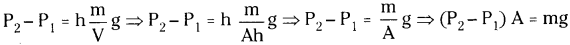Since F = PA and W = mg
We get F = W (Values of displaced liquid)

1. Here F’ is the force applied on the object and ‘W’ is the weight of the liquid.
2. So, the”force applied on the object by the liquid is equal to the weight of the displaced liquid.

9th Class Physical Science Textbook Page No. 155

Question 6.
Why does the stone lose weight when it is immersed?

• Suspend a stone from a spring balance. Note the reading of the spring balance.
• Take a beaker half-filled with water.
• Now immerse the stone in the water, note the reading of the spring balance.
• We notice that the stone, when immersed appears to lose some weight.
• The immersed stone appears to lose weight because the force of buoyancy.
• Thus the apparent loss of weight must be equal to the force of buoyancy acting on the immersed stone.

9th Class Physical Science Textbook Page No. 142

Question 7.
Let us suppose you have two blocks and you do not know what material they are made of. The volume of one block is 30 cm3 while the other is 60 cm3. The second block is heavier than the first. Based on this information, can you tell which of the two blocks is denser?
No, we cannot say which of the two blocks is denser, because any one of the quantity
i. e., either volume or weight must be same.

9th Class Physical Science Textbook Page No. 153

Question 8.
a) What would happen if Toricelli’s experiment is done on moon?
b) A stopper is inserted in the small hole of the glass tube of the mercury barometer below the top level of the mercury in it. What happens when you pull out the . stopper from the glass tube?
c) Why don’t we use water instead of mercury in Toricelli experiment? If we are ready to do this experiment, what length of tube is needed?
d) Find the weight of the atmosphere around the earth (take the radius of earth as 6400km)
a) If Toricelli’s experiment had been done on moon, the height of mercury column will be zero. Because there is no atmosphere on the moon.

b) The mercury level does not change, because there is vacuum above the mercury level. Hence no pressure is on the mercury. Also, the weight of mercury column must be equal to the air pressure above the reservoir. Hence there will be no change in the height of the mercury column.

c) We cannot use water instead of mercury in Toricelli experiment because, if we want to use water, we have to take the glass tube of length nearby above 10 m, which is inconvenient.
If water is taken,
P0 = ρhg
1 01 yin5
1.01 × 105 = 1 × 10³ × h × 9.8 ⇒ h = = 0.1030 × 10² = 10.3 m

d) Weight of atmosphere = Atmospheric pressure x Surface area of the earth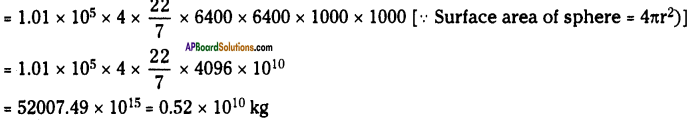9th Class Physical Science Textbook Page No. 157

Question 9.
a) Why is it easier for you to float in saltwater than in freshwater?
b) Why is there no horizontal buoyant force on a submerged body?
c) Two solid blocks of identical size are submerged in water. One block is iron and the other is aluminium. Upon which is the buoyant force greater?
d) A piece of iron when placed on a block of wood, this makes the wood to float lower in the water. If the iron piece is suspended beneath the wood block, would it float at the same depth? Or lower or higher?
a) Salt water is denser than freshwater.
b) Buoyant force is the upward force only. The body is submerged means, its weight is more than the buoyant force. Here there will be no horizontal buoyant force.
c) Buoyant force on iron block is more than that of aluminium block.
d) It floats on higher depth than in the first case.

9th Class Physical Science 9th Lesson Floating Bodies Activities

Activity – 1

Question 1.
Comparing density – relative density.
Take two test tubes of the same size and fill one to the brim with water and the other with oil.
a) Which will weigh more?
The test tube with oil will weigh more.

b) Which liquid is denser?
Oil is denser than water.
Take two equal sized blocks made of wood and rubber.

c) Which of these two blocks is heavier?
Wooden block is heavier.

d) Which one is denser?
Wooden block is denser than rubber.

Activity – 3

Question 2.
Do the objects denser than water float in it? Prove it with an activity.

• Collect some objects listed in the table below.
• Place them one by one in a glass of water and observe whether they sink or float in water.
• Record your observations in the table.

Observations: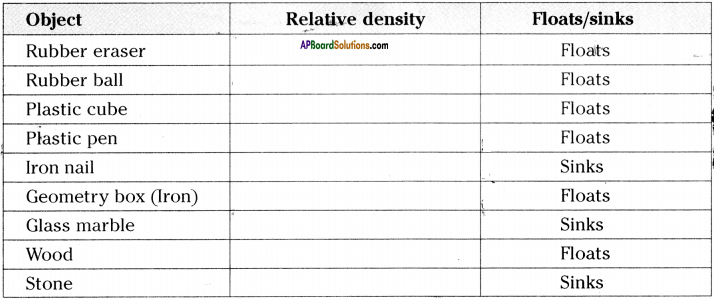• Find the relative densities of all the objects.
• We observe that some objects floats and some objects like iron nail, glass marble, stone sinks in water.
• Geometry box made of iron floats on water, though its relative density is greater than 1. This is due to its larger surface area.
• Geometry box made of iron floats on water though it is made up of a substance denser than water.
•  We can say that the floating or sinking of an object does not depend not only on its relative density, but also its surface area which displaces the water.

Activity – 4

Question 3.
Show that for a floating object, the weight of the object is equal to the weight of water displaced by it.• Take a beaker and weigh it. Note down its weight.
• Fill water in an overflow jar, wait until the water stops dripping from the outlet of the overflow jar.
• Now place the beaker below the outlet of the overflow jar.
• Take a wooden block, moisten it with water and gently drop it into the overflow jar.
• Water will flow out of the overflow jar and collects in the beaker kept under the overflow jar.
• Measure the weight of beaker with water.
• Subtract the weight of beaker from this. The value gives the weight of water displaced by wooden block. Note it.
• Now remove wooden block from the overflow jar, make it cry and weight.
• We can observe that the weight of wooden block is equal to weight of water displaced by it.
• Do the same experiment with some other substances and record your observations in the table.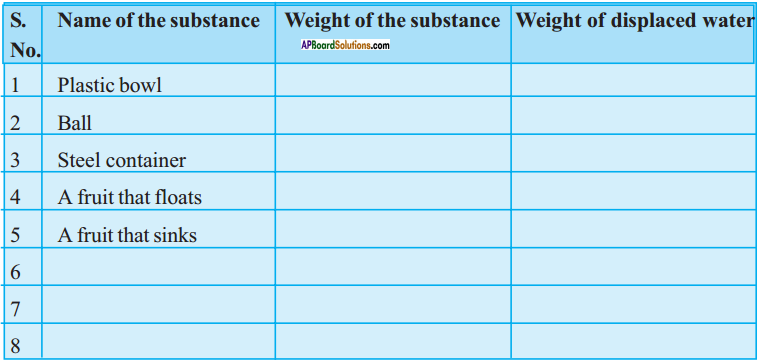Activity – 5

Question 4.
Explain how does metal aluminium floats on the water with an activity.

• Take a small sheet of aluminium foil.
• Fold it four or five times, pressing the foil tight after each fold.
• We can find that the aluminium foil will sink in the water.
• Now unfold the aluminium foil and make it as a small bowl.
• We can find that the bowl will float on the water.
• The metal bowl displace larger amount of water than a metal piece.
• Weight of the displaced water is more than the metal sheet.
• So, bowl will float on the water.

Activity – 6

Question 5.
Prove that the water exerts upward force on objects.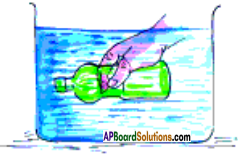1. Take an empty plastic bottle.
2. Put the cap on it tightly.
3. Place the bottle in a bucket of water.
4. The bottle will float.
5. Push the bottle into the water by your hand as shown in figure.
6. We feel some upward force.
7. Try to push it further down. We feel increase in the upward force.
8. Now release the bottle.
9. It bounce back to the surface of water.
10. Here the upward force of water a real, observable force.
11. This force acting on unit area of the surface of an object is called static pressure of the water.

Activity – 7

Question 6.
Describe an activity to observe the air pressure.1. Take a glass tumbler.
2. Stick some cotton at the bottom of it.
3. Immerse it inversely in water upto the bottom of the container as shown in figure.
4. Take out the tumbler from water.
5. We observe that the cotton attached at the bottom of the tumbler is not wet.
6. This is due to the force of air which is applied on water by the air present in the tumbler and stops water from entering the tumbler.
7. This force on unit area of water is the pressure of air.

Activity – 8

Question 7.
How can you measure the force of buoyancy and how much?
(OR)
Why does and stone lose weight when it is immersed in water?

• Suspend a stone from a spring balance.
• Note that the reading of the spring balance.
• The reading gives the weight of the stone.
• Take a beaker half filled with water.
• Now immerse the stone in the water.
• Note the reading of the spring balance.
• The reading gives the weight of the immersed stone.
• We may notice that the stone, when immersed, appears to lose some weight.
• The immersed stone appears to lose weight because the force of BUOYANCY, exerted on the stone by the water in the upward direction.
• Thus the apparent loss of weight must be equal to the force of buogancy acting on the immersed stone.
• The lose of weight of stone is equal to weight of the water displaced by the stone, this is the force of buoyancy.

Activity – 9

Question 8.
‘State and prove Archimedes principle of buoyancy.Archimedes principle :
Archimedes principle states that when a body is immersed in a fluid, it experiences an upward force of buoyancy equal to the weight of fluid displaced by the immersed portion of the body.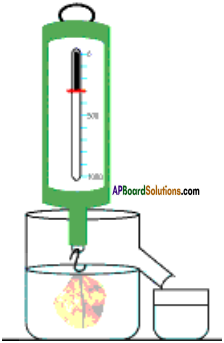Proof:

1. Suspend a stone from a spring balance.
2. Note the reading on the spring balance. This reading is the weight of the stone.
3. Take an overflow jar with water and place a graduated beaker below the beak as shown in the figure.
4. Now immerse the stone in the water and note the reading on the spring balance.
5. Measure the volume of the water that overflows into the graduated beaker.
6. The reading of the spring balance gives the weight of the immersed stone.
7. The beaker reading gives the volume of water displaced by the stone.
8. The difference in the two readings of spring balance gives the apparent loss of weight of the stone.
9. Now weigh the water in the graduated beaker.
10. We observe that the apparent loss of weight of the stone is equal to the weight of the water displaced by the stone.
11. Hence Archimedes principle is proved.